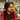LFU Cache

This is a follow up question to LRU cache which is covered Here

What’s an LFU Cache:

• A system to maintain a cache with in a limited amount of memory.
• If the memory is limited, obviously we’ll reach a point where there is no space left to store the cache item.
• So the least recently used (LFU) item is removed from the cache when the cache is full.
• We maintain a map to store the frequency of each key used in the cache and remove the key with the least frequency when the memory fills.
• We also maintain the position of the cache item which was used the earliest, just like we do in LRU Cache.
• In case there is a clash between the keys to be removed which will happen if multiple keys have the same least frequency, the least recently used key among the candidates is removed.
• So in a way, LFU required LRU cache implementation also.
• Valid operations on a cache system:

1. get(key)
2. put(key, value)

Example:

Cache capacity: 2
put(1,1)    //Cache: {1:1},         Frequency: {1:1}
put(2,2)    //Cache: {1:1, 2:2},    Frequency: {1:1, 2:1}
get(1)      //Cache: {1:1, 2:2},    Frequency: {1:2, 2:1},      Output: 1
put(3,3)    //Cache: {1:1, 3:3},    Frequency: {1:2, 3:1}
get(2)      //Cache: {1:1, 3:3},    Frequency: {1:2, 3:1},      Output: -1
get(3)      //Cache: {1:1, 3:3},    Frequency: {1:2, 3:2},      Output: 3
put(4,4)    //Cache: {3:3, 4:4},    Frequency: {3:2, 4:1} <-- Remove 1 because it was least recently used among 1 & 3
get(1)      //Cache: {3:3, 4:4}     Frequency: {3:3, 4:4},      Output: -1
get(3)      //Cache: {3:3, 4:4},    Frequency: {3:3, 4:1},      Output: 3
get(4)      //Cache: {3:3, 4:4},    Frequency: {3:3, 4:2},      Output: 4

Implementation

Here we will maintain a map frequency to maintain the frequency of each key used.
Also we’ll maintain an array history in which the items will be sorted according to their most recent occurrence.

var LFUCache = function (capacity) {
this.size = capacity
this.frequency = new Map()
this.map = new Map()
this.history = []
}

LFUCache.prototype.get = function (key) {
if (!this.map.has(key)) return -1

this.frequency.set(key, this.frequency.get(key) + 1)
this.updateHistory(key)
return this.map.get(key)
}

LFUCache.prototype.put = function (key, value) {
if (this.size == 0) return
if (this.map.has(key)) {
this.frequency.set(key, this.frequency.get(key) + 1)
this.updateHistory(key)
this.map.set(key, value)
} else {
if (this.map.size >= this.size) {
var leastFrequency = Math.min(...this.frequency.values())

var candidates = []
for (var frequencyKey of this.frequency.keys()) {
if (this.frequency.get(frequencyKey) == leastFrequency) {
candidates.push(frequencyKey)
}
}

var keyToDelete = candidates
if (candidates.length > 1) {
var leastIndex = Infinity
for (var candidate of candidates) {
var tempIndex = this.history.indexOf(candidate)
if (tempIndex != -1 && tempIndex < leastIndex) {
keyToDelete = candidate
leastIndex = tempIndex
}
}
this.history.splice(leastIndex, 1)
}

this.map.delete(keyToDelete)
this.frequency.delete(keyToDelete)
}

this.map.set(key, value)
this.frequency.set(key, 1)
this.updateHistory(key)
}
}
LFUCache.prototype.updateHistory = function (key) {
var index = this.history.indexOf(key)
if (index != -1) this.history.splice(index, 1)
this.history.push(key)
}

Execution:

var lfu = new LRUCache(2)

lfu.put(1, 1)
lfu.put(2, 2)
lfu.get(1)
lfu.put(3, 3)
lfu.get(2)
lfu.get(3)
lfu.put(4, 4)
lfu.get(1)
lfu.get(3)
lfu.get(4)Written by Gagandeep Rangi who likes to talk about himself in third person. Twitter Instagram